# CD Get System Dimensions VI

LabVIEW 2018 Control Design and Simulation Module Help

Edition Date: March 2018

Part Number: 371894J-01

»View Product InfoDownload Help (Windows Only)

Owning Palette: Model Information VIs

Requires: Control Design and Simulation Module

Obtains the number of inputs, outputs and, if applicable, the number of states of the system. Wire data to the State-Space Model input to determine the polymorphic instance to use or manually select the instance.

Use the pull-down menu to select an instance of this VI.

 Select an instance CD Get System Dimensions (State-Space)CD Get System Dimensions (Transfer Function)CD Get System Dimensions (Zero-Pole-Gain)

## CD Get System Dimensions (State-Space)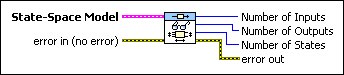State-Space Model contains a mathematical representation of and information about the system for which this VI obtains system dimensions.error in describes error conditions that occur before this node runs. This input provides standard error in functionality.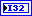Number of Inputs returns the number of inputs in the system model.Number of Outputs returns the number of outputs in the system model.Number of States returns the number of states in the system model.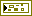error out contains error information. This output provides standard error out functionality.

## CD Get System Dimensions (Transfer Function)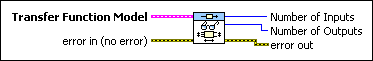Transfer Function Model contains a mathematical representation of and information about the system for which this VI obtains system dimensions.error in describes error conditions that occur before this node runs. This input provides standard error in functionality.Number of Inputs returns the number of inputs in the system model.Number of Outputs returns the number of outputs in the system model.error out contains error information. This output provides standard error out functionality.

## CD Get System Dimensions (Zero-Pole-Gain)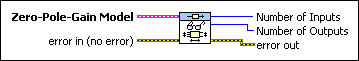Zero-Pole-Gain Model contains a mathematical representation of and information about the system for which this VI obtains system dimensions.error in describes error conditions that occur before this node runs. This input provides standard error in functionality.Number of Inputs returns the number of inputs in the system model.Number of Outputs returns the number of outputs in the system model.error out contains error information. This output provides standard error out functionality.